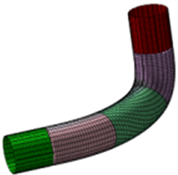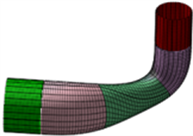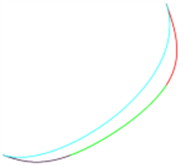﻿ 基于轴线光滑拼接的轴线异面管道拼接技术

基于轴线光滑拼接的轴线异面管道拼接技术Blending Technology of Tubes with Non-Coplanar Axes Based on Smooth Blending Axis

Abstract: The problem of smooth blending axes of non-coplanar tubes with cubic uniform B-spline curve is discussed. By the constraint of the control polygon, let B-spline curve pass through the vertices of the control polygon, and the curve is tangent to the edge of the control polygon. In this way, a piecewise cubic uniform B-spline curve can be constructed. Thus, this curve will be smoothly blended between axes of two non-coplanar tubes. And then, a smooth blending circular tube that takes B-spline curve as its axis is obtained.

1. 引言

$r\left(s\right)=\underset{i=0}{\overset{3}{\sum }}{B}_{i,3}\left(s\right){V}_{i}$

$\left\{\begin{array}{l}{N}_{0,3}=\frac{1}{6}\left(-{s}^{3}+3{s}^{2}-3s+1\right),\\ {N}_{1,3}=\frac{1}{6}\left(3{s}^{3}-6{s}^{2}+4\right),\\ {N}_{2,3}=\frac{1}{6}\left(-3{s}^{3}+3{s}^{2}+3s+1\right),\\ {N}_{3,3}=\frac{1}{6}{s}^{3}.\end{array}$

2. 构造基于轴线光滑拼接的轴线异面管道的光滑拼接管道

${\Phi }_{1}:\left\{\begin{array}{l}x={x}_{1}+{a}_{\text{1}}{N}_{11}\mathrm{cos}\phi +{a}_{\text{1}}{B}_{11}\mathrm{sin}\phi ,\\ y={y}_{1}+{b}_{1}s+{a}_{\text{1}}{N}_{12}\mathrm{cos}\phi +{a}_{\text{1}}{B}_{12}\mathrm{sin}\phi ,\\ z={a}_{\text{1}}{N}_{13}\mathrm{cos}\phi +{a}_{\text{1}}{B}_{13}\mathrm{sin}\phi .\end{array}$${\Phi }_{2}:\left\{\begin{array}{l}x={a}_{\text{2}}{N}_{21}\mathrm{cos}\phi +{a}_{\text{2}}{B}_{21}\mathrm{sin}\phi ,\\ y={y}_{2}+{a}_{\text{2}}{N}_{22}\mathrm{cos}\phi +{a}_{\text{2}}{B}_{22}\mathrm{sin}\phi ,\\ z={z}_{2}+{c}_{2}s+{a}_{\text{2}}{N}_{23}\mathrm{cos}\phi +{a}_{\text{2}}{B}_{23}\mathrm{sin}\phi .\end{array}\text{\hspace{0.17em}}\text{\hspace{0.17em}}\phi \in \left[0,2\text{π}\right]$

${L}_{1}:\left\{\begin{array}{l}x={x}_{1}+0\cdot s,\\ y={y}_{1}+{b}_{1}s,\\ z=0+0\cdot s,\end{array}$${L}_{2}:\left\{\begin{array}{l}x=0+0\cdot s,\\ y={y}_{2}+{b}_{\text{2}}\cdot s,\\ z={z}_{2}+{c}_{2}s.\end{array}$

${V}_{0}\left({x}_{0},{y}_{0},0\right),{V}_{1}\left({x}_{1},{y}_{1},0\right)$${L}_{1}$ 上的两个点， ${V}_{2}\left(0,{y}_{2},{z}_{2}\right),{V}_{1}\left(0,{y}_{3},{z}_{3}\right)$${L}_{2}$ 上的两个点，在 ${V}_{0}{V}_{1}$ 的反向延长线上取 ${V}_{-1}\left({x}_{1},{y}_{-1},0\right)$ ，使得 $|{V}_{1}-{V}_{0}|=|{V}_{0}-{V}_{-1}|$ ，在 ${V}_{2}{V}_{3}$ 的延长线上取 ${V}_{4}$ ，使 $|{V}_{2}-{V}_{3}|=|{V}_{3}-{V}_{4}|$ 。则以 ${V}_{1},{V}_{0},{V}_{1},{V}_{2},{V}_{3},{V}_{4}$ 为特征多边形的顶点的均匀三次B样条曲线通过顶点 ${V}_{0},{V}_{3}$ ，且与特征多边形的第一条边 ${V}_{0}{V}_{1}$ 与第三条边 ${V}_{2}{V}_{3}$ 相切。

3. 构造与轴线异面的圆管道Φ1与Φ2光滑拼接的三段光滑圆管道

$p\left(s,\phi \right)=\left\{\begin{array}{l}x\left(s\right)+a{N}_{1}\left(s\right)\mathrm{cos}\phi +a{B}_{1}\left(s\right)\mathrm{sin}\phi ,\\ y\left(s\right)+a{N}_{2}\left(s\right)\mathrm{cos}\phi +a{B}_{2}\left(s\right)\mathrm{sin}\phi ,\\ z\left(s\right)+a{N}_{3}\left(s\right)\mathrm{cos}\phi +a{B}_{3}\left(s\right)\mathrm{sin}\phi .\end{array}\text{\hspace{0.17em}}\text{\hspace{0.17em}}s\in \left[0,1\right],\phi \in \left(0,\text{π}\right)$

$N=\left({N}_{1}\left(s\right),{N}_{2}\left(s\right),{N}_{3}\left(s\right)\right),B=\left({B}_{1}\left(s\right),{B}_{2}\left(s\right),{B}_{3}\left(s\right)\right)$

${a}_{1}\ne {a}_{2}$ 时，再构造一个光滑拼接两个轴线异面的管道的某两个母线 ${r}_{\text{1}}\left(s\right)$${r}_{\text{2}}\left(s\right)$ 的B样条曲线 ${r}^{\prime }\left(s\right)$ ，则光滑拼接粗细不同的轴线异面的管道的拼接管道的表示式为

$p\left(s,\phi \right)=\left\{\begin{array}{l}x\left(s\right)+d\left(s\right){N}_{1}\left(s\right)\mathrm{cos}\phi +d\left(s\right){B}_{1}\left(s\right)\mathrm{sin}\phi ,\\ y\left(s\right)+d\left(s\right){N}_{2}\left(s\right)\mathrm{cos}\phi +d\left(s\right){B}_{2}\left(s\right)\mathrm{sin}\phi ,\\ z\left(s\right)+d\left(s\right){N}_{3}\left(s\right)\mathrm{cos}\phi +d\left(s\right){B}_{3}\left(s\right)\mathrm{sin}\phi .\end{array}\text{\hspace{0.17em}}\text{\hspace{0.17em}}s\in \left[0,1\right],\phi \in \left(0,\text{π}\right)$

4. 拼接实例Figure 1. Three-segment G1-blending diagram is same radiuses tubes whose axes are in non-coplaner take the B-spline curve as its axisFigure 2. Three-segment G1-blending diagram is different radiuses tubes whose axes are in non-coplaner take the B-spline curve as its axisFigure 3. Comparison of the smoothness of the same control vertex B-spline curve and Bézier curve

5. 结束语

 Hoffmann, E. and Hopcroft, J. (1986) Quadratic Blending Surfaces. Computer Aided Design, 18, 301-307.
https://doi.org/10.1016/0010-4485(86)90091-6

 Warren, J. (1989) Blending Algebraic Surfaces. ACM Transactions on Graphics, 8, 263-278.
https://doi.org/10.1145/77269.77270

 Cox, D., Sederberg, T.W. and Chen, F. (1998) The Moving Line Ideal Basis of Planar Rational Curves. Computer Aided Geometric Design, 15, 803-827.
https://doi.org/10.1016/S0167-8396(98)00014-4

 Chen, C.S., Chen, F. and Feng, Y.Y. (2001) Blending Quadric Surface with Piecewise Algebraic Surfaces. Graphics Models, 63, 212-223.
https://doi.org/10.1006/gmod.2001.0552

 雷娜, 崔丽, 伍铁如. 两个轴异面的管道拼接[J]. 吉林大学学报(理学版), 2002, 40(2): 138-140.

 Hartmann, E. (1995) Blending an Implicit with a Parametric Surface. CAGD, 12, 825-835.
https://doi.org/10.1016/0167-8396(95)00002-1

 Hartmann, E. (1998) Numerical Implicitization for Intersection and Gn-Continuous Blending of Surface. CAGD, 15, 377-397.
https://doi.org/10.1016/S0167-8396(97)00040-X

 Lei, N., Zhang, S., Dong, T. and Feng, G. (2005) The Existence and Expression of Osculatory Rational Interpolation. Journal of Information and Computational Science, 2, 493-500.

 白根柱. 两个轴异面圆管的光滑拼接问题[D]: [硕士学位论文]. 长春: 吉林大学, 2004.

 白根柱. 隐式代数曲面拼接[J]. 数学的实践与认识, 2006, 36(4): 274-277.

 Bai, G.Z., Wang, H. and Yin, Z.J. (2014) Employing Generalized Bezier Tube to Smoothly Blending Tubes Whose Axes Are Non-Coplanar. Applied Mechanics and Materials, 513-517, 2301-2306.
https://doi.org/10.4028/www.scientific.net/AMM.513-517.2301

 Wang, H. and Bai, G.Z. (2013) Employing Generalized Cylindrical Helicoid Tube to Smoothly Blending Tubes Whose Axes Are Non-Coplanar. Applied Mechanics and Materials, 380-384, 1750-1754.
https://doi.org/10.4028/www.scientific.net/AMM.380-384.1750

 Bai, G.Z. and Wu, Z. (2013) Multi-Degree Reduction Approximation of Bézier Curves by Subdivision Method. Intelligent Information Management Systems and Technologies, 9, 37-42.

 Bai, G.-Z., Liu, S.-Y., Wang, H., Wu, Z. and Yin, Z.-J. (2014) A Novel Method for Smooth Blending Cylindrical Sur-faces Whose Axes Are Non-Coplanar Based on Smooth Blending Axes. Applied Science, Materials Science and Information Tech-nologies in Industry, 2014, 687-691.
https://doi.org/10.4028/www.scientific.net/AMM.687-691.1470

 Bai, G.-Z., et al. (2014) A Novel Method for Smooth Blending Cylindrical Surfaces Whose Axes Are Non-Coplanar Based on Smooth Blending Axes. Applied Mechanics and Materials, 687-691, 1470-1473.
https://doi.org/10.4028/www.scientific.net/AMM.687-691.1470

 Bai, G.-Z., Wang, H., et al. (2014) Smoothly Blending of Two Elliptic Cylinders Whose Axes Are Non-Coplanar. Applied Mechanics and Materials, 309-312, 644-650.
https://doi.org/10.4028/www.scientific.net/AMM.644-650.309

 刘斯语, 白根柱. 利用推广的Hartmann方法光滑拼接两个异面直线[J]. 内蒙古民族大学学报(自然科学版), 2015(2): 102-104.

 邱梦鸾, 肖琳, 白根柱. 用单页双曲螺旋管实现两个轴异面管道的光滑拼接[J]. 重庆师范大学学报(自然科学版), 2016(2): 108-112.

Top